# Data structures#

Introduction to basic data structures handling.

## Introduction#

This is a getting started tutorial for Gammapy.

In this tutorial we will use the Third Fermi-LAT Catalog of High-Energy Sources (3FHL) catalog, corresponding event list and images to learn how to work with some of the central Gammapy data structures.

We will cover the following topics:

• Sky maps

• We will learn how to handle image based data with gammapy using a Fermi-LAT 3FHL example image. We will work with the following classes:

• Event lists

• We will learn how to handle event lists with Gammapy. Important for this are the following classes:

• Source catalogs

• We will show how to load source catalogs with Gammapy and explore the data using the following classes:

• Spectral models and flux points

• We will pick an example source and show how to plot its spectral model and flux points. For this we will use the following classes:

## Setup#

Important: to run this tutorial the environment variable `GAMMAPY_DATA` must be defined and point to the directory on your machine where the datasets needed are placed. To check whether your setup is correct you can execute the following cell:

```import astropy.units as u
from astropy.coordinates import SkyCoord
import matplotlib.pyplot as plt
```

## Check setup#

```from gammapy.utils.check import check_tutorials_setup

# %matplotlib inline

check_tutorials_setup()
```
```System:

python_executable      : /home/runner/work/gammapy-docs/gammapy-docs/gammapy/.tox/build_docs/bin/python
python_version         : 3.9.15
machine                : x86_64
system                 : Linux

Gammapy package:

version                : 1.0
path                   : /home/runner/work/gammapy-docs/gammapy-docs/gammapy/.tox/build_docs/lib/python3.9/site-packages/gammapy

Other packages:

numpy                  : 1.23.4
scipy                  : 1.9.3
astropy                : 5.1.1
regions                : 0.7
click                  : 8.1.3
yaml                   : 6.0
IPython                : 8.6.0
jupyterlab             : not installed
matplotlib             : 3.6.2
pandas                 : not installed
healpy                 : 1.16.1
iminuit                : 2.17.0
sherpa                 : 4.15.0
naima                  : 0.10.0
emcee                  : 3.1.3
corner                 : 2.2.1

Gammapy environment variables:

GAMMAPY_DATA           : /home/runner/work/gammapy-docs/gammapy-docs/gammapy-datasets/1.0
```

## Maps#

The `maps` package contains classes to work with sky images and cubes.

In this section, we will use a simple 2D sky image and will learn how to:

• Read sky images from FITS files

• Smooth images

• Plot images

• Cutout parts from images

```from gammapy.maps import Map

```

The image is a `WcsNDMap` object:

```print(gc_3fhl)
```
```WcsNDMap

geom  : WcsGeom
axes  : ['lon', 'lat']
shape : (400, 200)
ndim  : 2
unit  :
dtype : >i8
```

The shape of the image is 400 x 200 pixel and it is defined using a cartesian projection in galactic coordinates.

The `geom` attribute is a `WcsGeom` object:

```print(gc_3fhl.geom)
```
```WcsGeom

axes       : ['lon', 'lat']
shape      : (400, 200)
ndim       : 2
frame      : galactic
projection : CAR
center     : 0.0 deg, 0.0 deg
width      : 20.0 deg x 10.0 deg
wcs ref    : 0.0 deg, 0.0 deg
```

Let’s take a closer look a the `.data` attribute:

```print(gc_3fhl.data)
```
```[[0 0 0 ... 0 0 0]
[0 0 0 ... 0 0 0]
[0 0 0 ... 0 0 0]
...
[0 0 0 ... 0 0 1]
[0 0 0 ... 0 0 0]
[0 0 0 ... 0 0 1]]
```

That looks familiar! It just an ordinary 2 dimensional numpy array, which means you can apply any known numpy method to it:

```print(f"Total number of counts in the image: {gc_3fhl.data.sum():.0f}")
```
```Total number of counts in the image: 32684
```

To show the image on the screen we can use the `plot` method. It basically calls plt.imshow, passing the `gc_3fhl.data` attribute but in addition handles axis with world coordinates using astropy.visualization.wcsaxes and defines some defaults for nicer plots (e.g. the colormap ‘afmhot’):

```plt.figure()

gc_3fhl.plot(stretch="sqrt")
```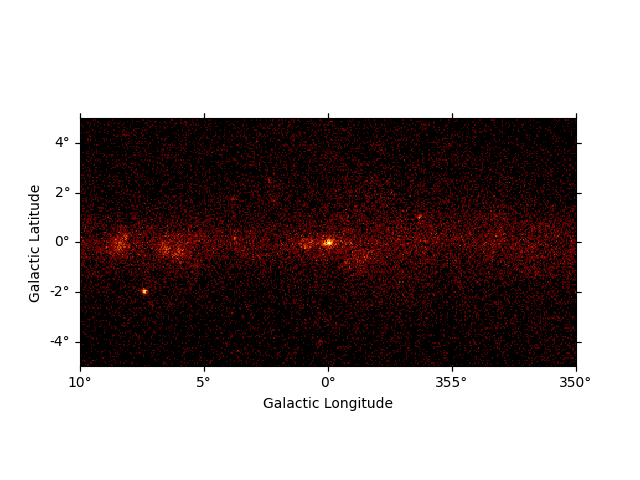```<WCSAxesSubplot: >
```

To make the structures in the image more visible we will smooth the data using a Gaussian kernel.

```plt.figure()
gc_3fhl_smoothed = gc_3fhl.smooth(kernel="gauss", width=0.2 * u.deg)

gc_3fhl_smoothed.plot(stretch="sqrt")
```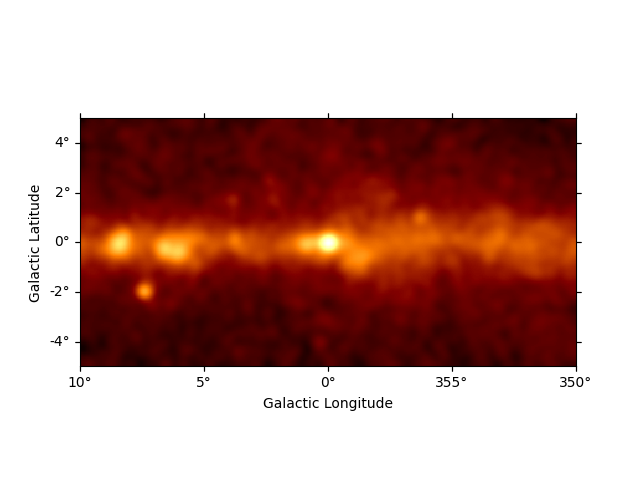```<WCSAxesSubplot: >
```

The smoothed plot already looks much nicer, but still the image is rather large. As we are mostly interested in the inner part of the image, we will cut out a quadratic region of the size 9 deg x 9 deg around Vela. Therefore we use `cutout` to make a cutout map:

```plt.figure()
# define center and size of the cutout region
center = SkyCoord(0, 0, unit="deg", frame="galactic")
gc_3fhl_cutout = gc_3fhl_smoothed.cutout(center, 9 * u.deg)

gc_3fhl_cutout.plot(stretch="sqrt")
```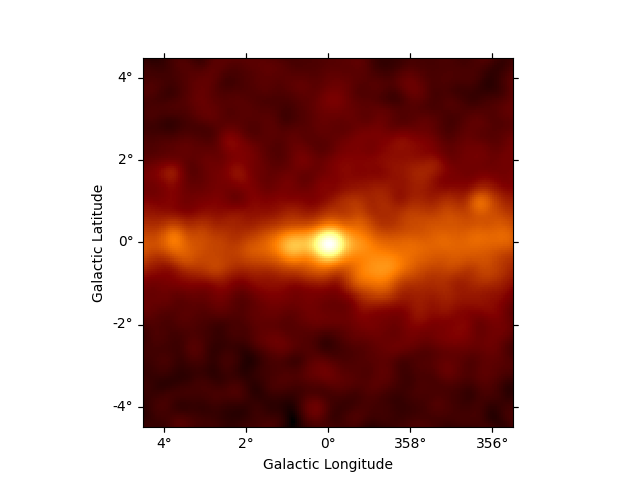```<WCSAxesSubplot: >
```

For a more detailed introduction to `maps`, take a look a the Maps notebook.

## Event lists#

Almost any high level gamma-ray data analysis starts with the raw measured counts data, which is stored in event lists. In Gammapy event lists are represented by the `EventList` class.

In this section we will learn how to:

• Read event lists from FITS files

• Access and work with the `EventList` attributes such as `.table` and `.energy`

• Filter events lists using convenience methods

Let’s start with the import from the `data` submodule:

```from gammapy.data import EventList
```

Very similar to the sky map class an event list can be created, by passing a filename to the `read()` method:

```events_3fhl = EventList.read("\$GAMMAPY_DATA/fermi-3fhl-gc/fermi-3fhl-gc-events.fits.gz")
```

This time the actual data is stored as an ` It can be accessed with ``.table`` attribute:

```print(events_3fhl.table)
```
```  ENERGY      RA       DEC         L      ... DIFRSP1 DIFRSP2 DIFRSP3 DIFRSP4
MeV       deg       deg        deg     ...
--------- --------- ---------- ---------- ... ------- ------- ------- -------
12186.642 260.45935 -33.553337  353.36273 ...     0.0     0.0     0.0     0.0
25496.598 261.37506 -34.395004  353.09607 ...     0.0     0.0     0.0     0.0
15621.498 259.56973 -33.409416  353.05673 ...     0.0     0.0     0.0     0.0
12816.32 273.95883 -25.340391    6.45856 ...     0.0     0.0     0.0     0.0
18988.387  260.8568 -36.355804  351.23734 ...     0.0     0.0     0.0     0.0
11610.23 266.15518 -26.224436  2.1986027 ...     0.0     0.0     0.0     0.0
13960.802 271.44742 -29.615316  1.6267247 ...     0.0     0.0     0.0     0.0
10477.372  266.3981  -28.96814  359.97003 ...     0.0     0.0     0.0     0.0
13030.88 271.70428 -20.632627    9.59348 ...     0.0     0.0     0.0     0.0
11517.904 265.00894 -30.065119  358.40112 ...     0.0     0.0     0.0     0.0
...       ...        ...        ... ...     ...     ...     ...     ...
387834.72  270.3779  -21.56711   8.171749 ...     0.0     0.0     0.0     0.0
20559.74  268.5538 -26.345692   3.200638 ...     0.0     0.0     0.0     0.0
27209.146 266.59344  -30.52607  358.72775 ...     0.0     0.0     0.0     0.0
13911.061 269.30997 -27.239439  2.7684028 ...     0.0     0.0     0.0     0.0
13226.425 265.16287 -27.344238  0.7796942 ...     0.0     0.0     0.0     0.0
17445.463 266.63342 -28.807201 0.21464892 ...     0.0     0.0     0.0     0.0
13133.864 270.42474 -22.651058   7.251185 ...     0.0     0.0     0.0     0.0
32095.705  266.0002  -29.77206   359.1034 ...     0.0     0.0     0.0     0.0
18465.783 266.39728 -29.105953  359.85202 ...     0.0     0.0     0.0     0.0
14457.25 262.72217 -34.388405   353.7184 ...     0.0     0.0     0.0     0.0
Length = 32843 rows
```

You can do len over event_3fhl.table to find the total number of events.

```print(len(events_3fhl.table))
```
```32843
```

And we can access any other attribute of the `Table` object as well:

```print(events_3fhl.table.colnames)
```
```['ENERGY', 'RA', 'DEC', 'L', 'B', 'THETA', 'PHI', 'ZENITH_ANGLE', 'EARTH_AZIMUTH_ANGLE', 'TIME', 'EVENT_ID', 'RUN_ID', 'RECON_VERSION', 'CALIB_VERSION', 'EVENT_CLASS', 'EVENT_TYPE', 'CONVERSION_TYPE', 'LIVETIME', 'DIFRSP0', 'DIFRSP1', 'DIFRSP2', 'DIFRSP3', 'DIFRSP4']
```

For convenience we can access the most important event parameters as properties on the `EventList` objects. The attributes will return corresponding Astropy objects to represent the data, such as `Quantity`, `SkyCoord` or `Time` objects:

```print(events_3fhl.energy.to("GeV"))
```
```[12.186643 25.4966   15.621499 ... 32.095707 18.465784 14.457251] GeV
```
```print(events_3fhl.galactic)
```
```<SkyCoord (Galactic): (l, b) in deg
[(353.36228879,  1.75408483), (353.09562941,  0.6522806 ),
(353.05628243,  2.44528685), ..., (359.10295505, -0.1359316 ),
(359.85157506, -0.08269984), (353.71795506, -0.26883694)]>
```
```print(events_3fhl.time)
```
```[54682.82946153 54682.89243456 54682.89709472 ... 57236.75267735
57233.37455141 57233.44802852]
```

In addition `EventList` provides convenience methods to filter the event lists. One possible use case is to find the highest energy event within a radius of 0.5 deg around the vela position:

```# select all events within a radius of 0.5 deg around center
from gammapy.utils.regions import SphericalCircleSkyRegion

region = SphericalCircleSkyRegion(center, radius=0.5 * u.deg)
events_gc_3fhl = events_3fhl.select_region(region)

# sort events by energy
events_gc_3fhl.table.sort("ENERGY")

# and show highest energy photon
print("highest energy photon: ", events_gc_3fhl.energy[-1].to("GeV"))
```
```highest energy photon:  1917.85925 GeV
```

### Exercises#

• Make a counts energy spectrum for the galactic center region, within a radius of 10 deg.

## Source catalogs#

Gammapy provides a convenient interface to access and work with catalog based data.

In this section we will learn how to:

• Load builtins catalogs from `catalog`

• Sort and index the underlying Astropy tables

• Access data from individual sources

Let’s start with importing the 3FHL catalog object from the `catalog` submodule:

```from gammapy.catalog import SourceCatalog3FHL
```

First we initialize the Fermi-LAT 3FHL catalog and directly take a look at the `.table` attribute:

```fermi_3fhl = SourceCatalog3FHL()
print(fermi_3fhl.table)
```
```   Source_Name     RAJ2000  DEJ2000  ... Redshift     NuPeak_obs
deg      deg    ...                  Hz
------------------ -------- -------- ... -------- ------------------
3FHL J0001.2-0748    0.3107  -7.8075 ...       --  306196370000000.0
3FHL J0001.9-4155    0.4849 -41.9303 ...       -- 6309576500000000.0
3FHL J0002.1-6728    0.5283 -67.4825 ...       -- 4466832000000000.0
3FHL J0003.3-5248    0.8300 -52.8150 ...       --       7.079464e+16
3FHL J0007.0+7303    1.7647  73.0560 ...       --                 --
3FHL J0007.9+4711    1.9931  47.1920 ...   0.2800 2511884200000000.0
3FHL J0008.4-2339    2.1243 -23.6514 ...   0.1470  524807800000000.0
3FHL J0009.1+0628    2.2874   6.4814 ...       --  663742400000000.0
3FHL J0009.4+5030    2.3504  50.5049 ...       -- 1412536400000000.0
3FHL J0009.7-4319    2.4450 -43.3195 ...       --                 --
...      ...      ... ...      ...                ...
3FHL J2347.9-1630  356.9978 -16.5106 ...   0.5760    9332549000000.0
3FHL J2350.5-3006  357.6354 -30.1070 ...   0.2237 3981075200000000.0
3FHL J2351.5-7559  357.8926 -75.9890 ...       --                 --
3FHL J2352.1+1753  358.0415  17.8865 ...       -- 1737799900000000.0
3FHL J2356.2+4035  359.0746  40.5985 ...   0.1310 6309576500000000.0
3FHL J2357.4-1717  359.3690 -17.2996 ...       --       8.912525e+16
3FHL J2358.4-1808  359.6205 -18.1408 ...       --                 --
3FHL J2358.5+3829  359.6266  38.4963 ...       --                 --
3FHL J2359.1-3038  359.7760 -30.6397 ...   0.1650       2.818388e+17
3FHL J2359.3-2049  359.8293 -20.8256 ...   0.0960 4073799600000000.0
Length = 1556 rows
```

This looks very familiar again. The data is just stored as an `Table` object. We have all the methods and attributes of the `Table` object available. E.g. we can sort the underlying table by `Signif_Avg` to find the top 5 most significant sources:

```# sort table by significance
fermi_3fhl.table.sort("Signif_Avg")

# invert the order to find the highest values and take the top 5
top_five_TS_3fhl = fermi_3fhl.table[::-1][:5]

# print the top five significant sources with association and source class
print(top_five_TS_3fhl[["Source_Name", "ASSOC1", "ASSOC2", "CLASS", "Signif_Avg"]])
```
```   Source_Name               ASSOC1           ...  CLASS  Signif_Avg
------------------ -------------------------- ... ------- ----------
3FHL J0534.5+2201  Crab Nebula                ... PWN        168.641
3FHL J1104.4+3812  Mkn 421                    ... BLL        144.406
3FHL J0835.3-4510  PSR J0835-4510             ... PSR        138.801
3FHL J0633.9+1746  PSR J0633+1746             ... PSR         99.734
3FHL J1555.7+1111  PG 1553+113                ... BLL         94.411
```

If you are interested in the data of an individual source you can access the information from catalog using the name of the source or any alias source name that is defined in the catalog:

```mkn_421_3fhl = fermi_3fhl["3FHL J1104.4+3812"]

# or use any alias source name that is defined in the catalog
mkn_421_3fhl = fermi_3fhl["Mkn 421"]
print(mkn_421_3fhl.data["Signif_Avg"])
```
```144.40611
```

### Exercises#

• Try to load the Fermi-LAT 2FHL catalog and check the total number of sources it contains.

• Select all the sources from the 2FHL catalog which are contained in the Galactic Center region. The methods `contains()` and `positions` might be helpful for this. Add markers for all these sources and try to add labels with the source names.

• Try to find the source class of the object at position ra=68.6803, dec=9.3331

## Spectral models and flux points#

In the previous section we learned how access basic data from individual sources in the catalog. Now we will go one step further and explore the full spectral information of sources. We will learn how to:

• Plot spectral models

• Compute integral and energy fluxes

• Read and plot flux points

```crab_3fhl = fermi_3fhl["Crab Nebula"]
crab_3fhl_spec = crab_3fhl.spectral_model()
print(crab_3fhl_spec)
```
```PowerLawSpectralModel

type      name     value         unit      ... max frozen is_norm link
-------- --------- ---------- -------------- ... --- ------ ------- ----
spectral     index 2.2202e+00                ... nan  False   False
spectral amplitude 1.7132e-10 cm-2 GeV-1 s-1 ... nan  False    True
spectral reference 2.2726e+01            GeV ... nan   True   False
```

The `crab_3fhl_spec` is an instance of the `PowerLaw2SpectralModel` model, with the parameter values and errors taken from the 3FHL catalog.

Let’s plot the spectral model in the energy range between 10 GeV and 2000 GeV:

```plt.figure()
ax_crab_3fhl = crab_3fhl_spec.plot(energy_bounds=[10, 2000] * u.GeV, energy_power=0)
```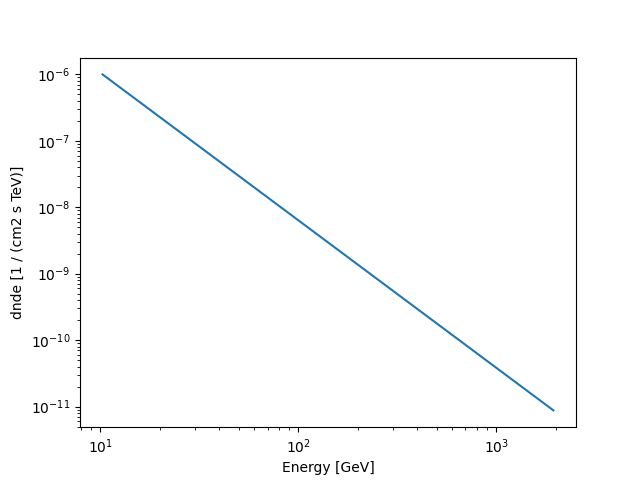We assign the return axes object to variable called `ax_crab_3fhl`, because we will re-use it later to plot the flux points on top.

To compute the differential flux at 100 GeV we can simply call the model like normal Python function and convert to the desired units:

```print(crab_3fhl_spec(100 * u.GeV).to("cm-2 s-1 GeV-1"))
```
```6.3848912826152664e-12 1 / (cm2 GeV s)
```

Next we can compute the integral flux of the Crab between 10 GeV and 2000 GeV:

```print(
crab_3fhl_spec.integral(energy_min=10 * u.GeV, energy_max=2000 * u.GeV).to(
"cm-2 s-1"
)
)
```
```8.67457342435522e-09 1 / (cm2 s)
```

We can easily convince ourself, that it corresponds to the value given in the Fermi-LAT 3FHL catalog:

```print(crab_3fhl.data["Flux"])
```
```8.658909145253801e-09 1 / (cm2 s)
```

In addition we can compute the energy flux between 10 GeV and 2000 GeV:

```print(
crab_3fhl_spec.energy_flux(energy_min=10 * u.GeV, energy_max=2000 * u.GeV).to(
"erg cm-2 s-1"
)
)
```
```5.311489174710791e-10 erg / (cm2 s)
```

Next we will access the flux points data of the Crab:

```print(crab_3fhl.flux_points)
```
```FluxPoints
----------

geom                   : RegionGeom
axes                   : ['lon', 'lat', 'energy']
shape                  : (1, 1, 5)
quantities             : ['norm', 'norm_errp', 'norm_errn', 'norm_ul', 'sqrt_ts', 'is_ul']
ref. model             : pl
n_sigma                : 1
n_sigma_ul             : 2
sqrt_ts_threshold_ul   : 1
sed type init          : flux
```

No we can check again the underlying astropy data structure by accessing the `.table` attribute:

```print(crab_3fhl.flux_points.to_table(sed_type="dnde", formatted=True))
```
``` e_ref    e_min   e_max         dnde      ...     dnde_ul     sqrt_ts is_ul
GeV      GeV     GeV    1 / (cm2 GeV s) ... 1 / (cm2 GeV s)
-------- ------- -------- --------------- ... --------------- ------- -----
14.142  10.000   20.000       5.120e-10 ...             nan 125.157 False
31.623  20.000   50.000       7.359e-11 ...             nan  88.715 False
86.603  50.000  150.000       9.024e-12 ...             nan  59.087 False
273.861 150.000  500.000       7.660e-13 ...             nan  33.076 False
1000.000 500.000 2000.000       4.291e-14 ...             nan  15.573 False
```

Finally let’s combine spectral model and flux points in a single plot and scale with `energy_power=2` to obtain the spectral energy distribution:

```plt.figure()
ax = crab_3fhl_spec.plot(energy_bounds=[10, 2000] * u.GeV, sed_type="e2dnde")
crab_3fhl.flux_points.plot(ax=ax, sed_type="e2dnde")

plt.show()
```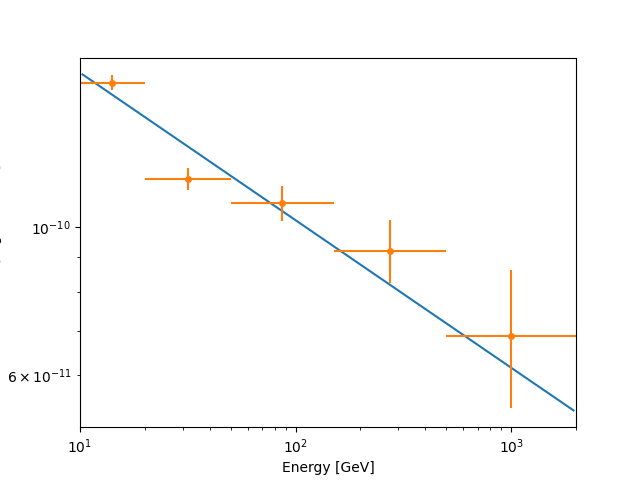### Exercises#

• Plot the spectral model and flux points for PKS 2155-304 for the 3FGL and 2FHL catalogs. Try to plot the error of the model (aka “Butterfly”) as well.

## What next?#

This was a quick introduction to some of the high level classes in Astropy and Gammapy.